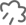# 整数加法运算定律推广到小数，整数加法运算定律推广到小数反思？

author 2023-07-19

# 从整数加法运算定律到小数：发现与反思## 1. 小数加法的交换律

1.2 + 2.3 = 3.5

2.3 + 1.2 = 3.5

1.25 + 0.75 = 2.00

0.75 + 1.25 = 2.00

## 2. 小数加法的结合律

1.2 + (2.3 + 3.4) = 1.2 + 5.7 = 6.9

(1.2 + 2.3) + 3.4 = 3.5 + 3.4 = 6.9

## 结论